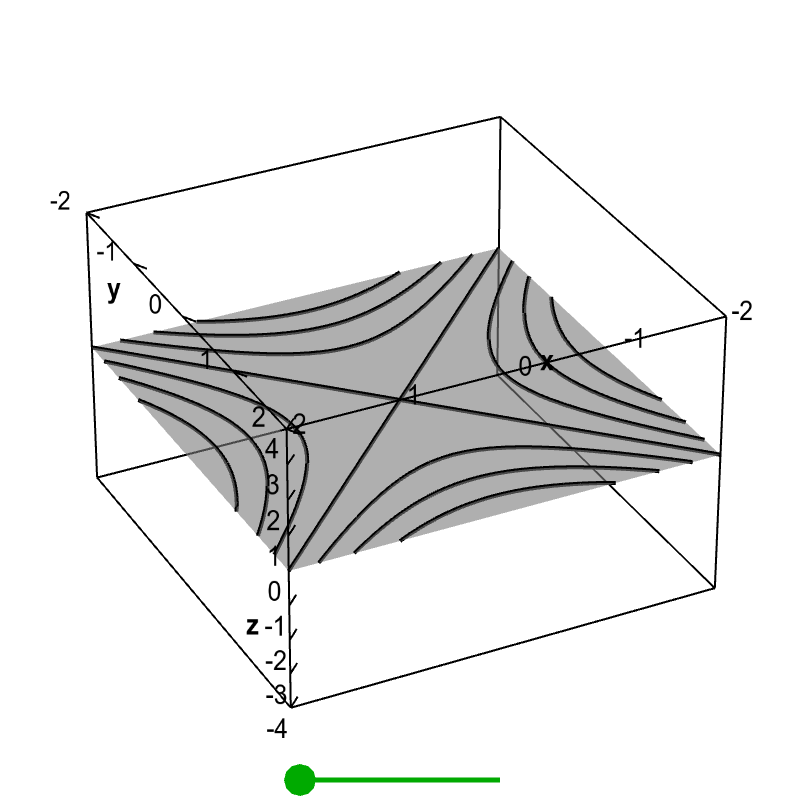# Math Insight

### Applet: Level curves of a hyperbolic paraboloidWhen the green point on the slider is to the left, as it is in the default view, the figure shows a standard level curve plot of $f(x,y)=x^2-y^2$, though it is floating in a three dimensional space. When you drag the green point to the right, each level curve $f(x,y)=c$ moves to the height $z=c$, so that they are in the same position as in the graph of $z=f(x,y)$. In this way, the figure demonstrates the correspondence between the level curve plot and the graph of the function.